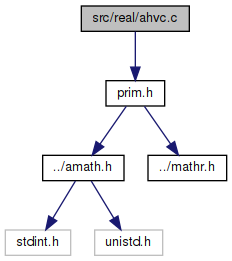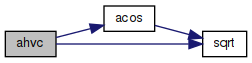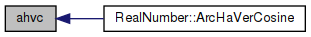amath  1.8.5 Simple command line calculator
ahvc.c File Reference

Inverse haversed cosine. More...

`#include "prim.h"`
Include dependency graph for ahvc.c:Go to the source code of this file.

## Functions

double ahvc (double x)
Inverse haversed cosine. More...

## Detailed Description

Inverse haversed cosine.

Definition in file ahvc.c.

## ◆ ahvc()

 double ahvc ( double x )

Inverse haversed cosine.

Definition at line 40 of file ahvc.c.

References acos(), and sqrt().

Referenced by RealNumber::ArcHaVerCosine().

41 {
42  double a = sqrt(x);
43  double b = acos(a);
44  double c = 2.0 * b;
45  return c;
46 }
double acos(double x)
Inverse cosine function.
Definition: acos.c:92
double sqrt(double x)
Square root function.
Definition: sqrt.c:119
Here is the call graph for this function:Here is the caller graph for this function: